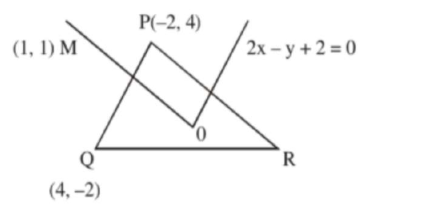# In a triangleQuestion:

In a triangle $\mathrm{PQR}$, the co-ordinates of the points $\mathrm{P}$ and $\mathrm{Q}$ are $(-2,4)$ and $(4,-2)$ respectively. If the equation of the perpendicular bisector of $\mathrm{PR}$ is $2 x-y+2=0$, then the centre of the circumcircle of the $\triangle \mathrm{PQR}$ is :

1. (1) $(-1,0)$

2. (2) $(-2,-2)$

3. (3) $(0.2)$

4. (4) $(1,4)$

Correct Option: , 2

Solution:Equation of perpendicular bisector of $P R$ is

$y=x$

Solving with $2 \mathrm{x}-\mathrm{y}+2=0$ will give

$(-2,2)$